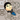# Suppress Error of type level programming of TypeScript

2019/04/15

When type level programming, various errors occur.So we need to suppress it.
I checked with v3.4.2.

## Suppress type constraint error

There are operations in TS that can only be of the type that satisfies the condition. Here are some examples.

type F<T extends any[]> = T;
type X = F<A>;// A require to extends any[]
type Y = B["x"]// B require object type and have roperty named x

However, even if it is known that the condition is satisfies, an error may occur.
At such time, let’s define and use the following Cast type.
This is used by coding Cast<T, P> when it is known that T extends P.

type Cast<T, P> = T extends P ? T : P;

example

type F<T> = "x" extends keyof T ? T : { x: 1 };
type G<T> = F<T>["x"];

We know that the result of F<T> have roperty named x.But an error occurs.
You solve it by coding as follows.

type G<T> = Cast<F<T>, { x: any }>["x"];

## Suppress Type instantiation is excessively deep and possibly infinite.

There are two types of errors.

1. When type parameter does not exist
2. When type parameter does exist

1 can not be suppressed because it exceeds the limit of recursion.
However, 2 can be suppressed.

When F and G are complex type constructors including recursion etc, an error will occur if coding the following:

type X<T> = G<F<T>>;

However, even if G is not so complicated, errors may occur.(need investigation)
At this time, it is solved by coding as follows.

type X<T> = G<F<T> extends infer A ? A : never>;

Complex type constructors often can not be used to infer type checking, so they are often used with Cast.

type X<T> = G<F<T> extends infer A ? Cast<A, any[]> : never>;

Checking became severe in TS3.4, and it became mandatory to use this technique.
issue

An example where an error occurs.

type Head<T extends any[], D = never> = T extends [infer X, ...any[]] ? X : D;
type Tail<T extends any[]> = ((...x: T) => void) extends ((x: any, ...xs: infer XS) => void) ? XS : never
type Cons<X, XS extends any[]> = ((h: X, ...args: XS) => void) extends ((...args: infer R) => void) ? R : [];
type Reverse<L extends any[], X extends any[] = []> = {
1: X, 0: Reverse<Tail<L>, Cons<Head<L>, X>>
}[L extends [] ? 1 : 0];

type X<T extends any[]> = Reverse<Reverse<T>>;

Solution.

type X<T extends any[]> = Reverse<Reverse<T> extends infer A ? Cast<A, any[]> : never>;

Examples of 1.

type X = Reverse<[1, 1, 1, 1, 1, 1, 1, 1, 1, 1, 1, 1, 1, 1, 1, 1, 1, 1, 1, 1, 1, 1, 1, 1, 1, 1, 1, 1, 1, 1, 1, 1, 1, 1, 1, 1, 1, 1, 1, 1, 1, 1, 1, 1]>;

This needs to stop dealing with large sized data, or be devised to reduce type recursion.Web Developer.